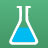cancel
Showing results for
Did you mean:New Contributor

## Acid Base Neutralization with weak acid or unequal moles of acid and base?

Trial 1: at 25 degrees, 4 grams of NaOH was dissolved in 100 mL of water. The mixture temperature was recorded as 38 degrees.

Trial 2: At 25 degrees 50 mL of 2 M NaOH was combined with 50 mL of 2 M HCl. The mixture temperature was recorded as 71 degrees.

1. If a neutralization similar to trial 2 were performed with the same molar values of naoh and ACETIC ACID, which if the following results is most likely to occur?

A) a higher temperature due to a larger |delta H|

B)a lower temperature due to a larger |delta H|

C) a higher temperature due to a smaller |delta H|

D) a lower temperature due to a smaller |delta H|

2. If trial 2 were performed with 25 mL of NaOH insetad of 50 mL the resulting soluion would have

A) pH<1

B) T= 71 degrees

C) [h3O+]=[OH-]

D) the same delta H

I know the answer should be A because you have more acid, but why are B and D not true specifically?

3. The freezing point of the mixed solution in Trial 2 is expected to be

A) lower than the freezing point temperatures of the initial acid and base solutions

B) the same as the freezing point temperatures of the initial acid and base solutions

C) higher than the freezing point temperatures of the initial acid and base solutions

D) lower than the freezing point temperatures of the initial acid and base solutions but higher than their melting point temperatures

I know D is not correct. I think that because the mixing temperature of trial 2 was 71 degrees, the solution has stronger IMFs than the individual acid base solutions, which would make "C" correct. Or does it have something to do with colligative properties?

Tags (1)